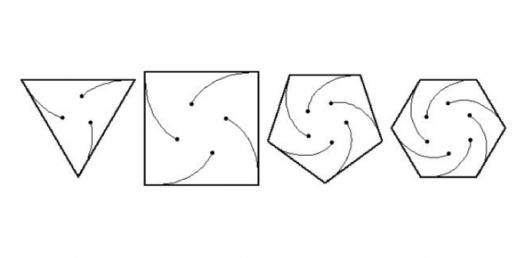# What Do You Know About The Mice Problem?

Approved & Edited by ProProfs Editorial Team
The editorial team at ProProfs Quizzes consists of a select group of subject experts, trivia writers, and quiz masters who have authored over 10,000 quizzes taken by more than 100 million users. This team includes our in-house seasoned quiz moderators and subject matter experts. Our editorial experts, spread across the world, are rigorously trained using our comprehensive guidelines to ensure that you receive the highest quality quizzes.
A
Community Contributor
Quizzes Created: 145 | Total Attempts: 38,511
Questions: 10 | Attempts: 126SettingsOften called the mice problem, this is a mathematical problem in which a predefined number of mice (or any other animal) are placed at specific points of a regular polygon. In the mice problem, each mouse moves towards another mouse in a certain direction and when they all meet, the problem is determined.

• 1.

### What is the mice problem also called?

• A.

Butterfly problem

• B.

Cat problem

• C.

Fly problem

• D.

Beetle problem

D. Beetle problem
Explanation
The correct answer is "Beetle problem" because the phrase "mice problem" is another term commonly used to refer to an infestation of beetles. This term is often used colloquially to describe a situation where there is a large number of beetles causing damage or nuisance in a particular area.

Rate this question:

• 2.

### How many mice are in the vertices of a square?

• A.

1

• B.

2

• C.

3

• D.

4

D. 4
Explanation
A square has four vertices, one at each corner. Therefore, there are four mice in the vertices of a square.

Rate this question:

• 3.

### Where do the mice meet in the polygon?

• A.

Center

• B.

Right corner

• C.

Upper layer

• D.

Left side

A. Center
Explanation
The correct answer is "Center" because in a polygon, the center is the point that is equidistant from all the vertices. Therefore, it is the meeting point for all the mice in the polygon.

Rate this question:

• 4.

### Which side of the regular polygon is the mice placed?

• A.

Corner

• B.

Center

• C.

Left side

• D.

Right side

A. Corner
Explanation
The mice are placed on the corner of the regular polygon because they are not in the center, left side, or right side.

Rate this question:

• 5.

### What kind of traces do the mice form?

• A.

Straight lines

• B.

Longitudinal spirals

• C.

Logarithmic spirals

• D.

Zigzag lines

C. Logarithmic spirals
• 6.

### Where does the mice move to after placing them?

• A.

Towards the side of the polygon

• B.

Towards its immediate neighbour

• C.

Towards the upper part

• D.

Towards the lower part

B. Towards its immediate neighbour
Explanation
The mice move towards their immediate neighbor because the question implies that the mice are placed within a polygon. In a polygon, each point is connected to its adjacent points, forming a network of interconnected sides. Therefore, when the mice are placed, they will naturally move towards their immediate neighbor, which is the point directly connected to their current position.

Rate this question:

• 7.

### To what direction does the mouse move?

• A.

On a straight line

• B.

Regular directions

• C.

Random directions

• D.

Clockwise

• E.

Anticlockwise

• F.

Anticlockwise or clockwise

F. Anticlockwise or clockwise
Explanation
The mouse can move in either an anticlockwise or clockwise direction. This means that it can move either to the left or to the right on a straight line, or it can move in a regular or random direction.

Rate this question:

• 8.

### What do we call the curve formed after the connection of the mice?

• A.

Wire

• B.

Stripe

• C.

Loop

• D.

Whirl

D. Whirl
Explanation
The curve formed after connecting the mice is called a whirl. A whirl refers to a spiral or circular movement, which accurately describes the shape created by the connection of the mice.

Rate this question:

• 9.

### When do we determine the result?

• A.

• B.

After the collision of the mice

• C.

After 20 mins into the problem

• D.

At the edge of the shape

B. After the collision of the mice
Explanation
The correct answer is "After the collision of the mice" because the question is asking about when the result is determined. The collision of the mice is a significant event that would likely lead to a result or outcome. Therefore, it makes sense that the result would be determined after this event occurs. The other options mentioned in the question, such as "At the head start," "After 20 mins into the problem," and "At the edge of the shape," are not relevant to determining the result and are therefore incorrect.

Rate this question:

• 10.

### At what rate does the mouse move?

• A.

Fast rate

• B.

Constant rate

• C.

Variable rate

• D.

Predertermined rate

B. Constant rate
Explanation
The correct answer is constant rate because the question asks about the rate at which the mouse moves. A constant rate means that the mouse moves at the same speed throughout, without any variations or changes in its speed.

Rate this question:

Related TopicsBack to top